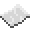# math.log

math.log
Function
Syntax
math.log(
• num : number
)

Returns number
API math
Source Lua (source)

Returns the natural logarithms of a number.ExampleGet natural logarithms
Get the natural logarithms of 5 and print it
Code
```<nowiki>
print(math.log(5))
</nowiki>
```
Output 1.6094379124341